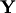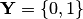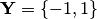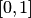# Probabilistic Classification

In probabilistic classification problems, the label spaceis finite and Forecaster is required to output a probability measure on. In binary classification problems, the size ofis 2; usually,or, and a forecast can be interpreted as a number in the interval(the predicted probability of the outcome 1).

An example of forecasting strategies for probabilistic classification is various Venn predictors.